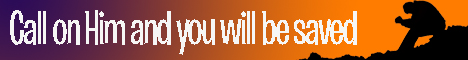Note:  Do not rely on this information. It is very old.

# Mathematics

Mathematics is the science of number. Any quantity requires for its complete expression a representation of its magnitude; if it only requires this, it is termed a number, and the study of such representation is termed notation. Most quantities occurring in nature are, however, more complex; not only do they require magnitude, but also a knowledge of the standard of comparison. A simple length, for instance, requires a number and a unit, as three feet, thirty-six inches, or one yard. Here the same physical quantity is represented by entirely different numbers - 3, 36, and 1 respectively

- there being in each case different standards of comparison - viz. the foot, the inch, and the yard. The correct distinction may at once be inferred between pure and applied mathematics. The former studies pure number and the relations between numbers; the latter involves the use of various units or standards of comparison. But, on the other hand, the study of pure number is in many cases simplified by attaching meanings to the quantities involved, beyond what they actually hold. The product of two numbers, for example, is often more clearly understood when the numbers themselves are assumed to represent lengths, and their product an area. On this account, pure mathematics deals with elementary units to an indefinite extent, and applied mathematics is made to cover chiefly those branches of the science that involve more intricate units, such as those of force or energy. Numbers are conveniently represented by lines, and investigation by such graphical means is the principle of geometry. This branch may involve a study of space-relationships that are apparently independent of magnitude, but even in such cases there is a connection, though its nature may be so intricate as to remain unexplained. When numbers are represented for brevity 'by symbols, such as letters of the alphabet, combined with other symbols, denoting operations performed on these numbers, such as raising to given powers, or the extracting of given roots, we enter the domain of algebra. The properties of points, lines, areas, and volumes in space may be investigated entirely by means of their position and magnitude, without the use of notation system; this is pure geometry. Or they may be investigated with the aid of ordinary numbers, or symbols representing ordinary numbers; this is analytical geometry. These properties are specially studied in the case of triangles and polygons in connection with angular measurement, and lead to trigonometry, plane, in the case of figures lying in one plane, spherical in the case of curvilinear figures that lie on the surfaces of spheres. The infinitesimal calculus deals with small increments in variable quantities, and must be regarded solely as a method, though an exceedingly powerful one, for prosecuting algebraic research; its two chief branches, the differential and the integral calculus, are converse in their nature, as multiplication and division, though it is nearer to the truth to define their difference as being that between analysis and synthesis. Applied mathematics is many-headed; all branches of natural philosophy require applications of mathematics, and an advance of knowledge in any branch may be often initiated by purely mathematical reasoning. The most direct applications are to the study of force in dynamics, while this subject again is continually introduced in physical questions, such as those on light, heat, magnetism, electricity, chemistry, or astronomy; but the connection between these sciences is too intimate to allow us to further'subdivide the subject of applied mathematics.